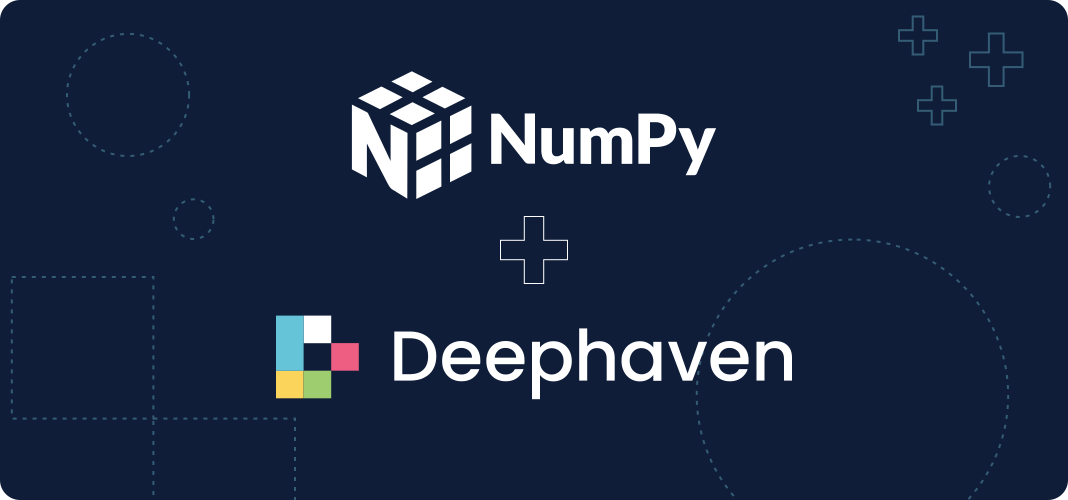# Use NumPy and real-time data with Deephaven

Leverage NumPy with Deephaven because large tables require faster processing## NumPy comes standard with Deephaven Core​

Deephaven leverages NumPy because large tables require faster processing. The vast majority of NumPy is implemented in C and wrapped in Python, which outperforms standard Python code. Furthermore, NumPy’s array operations translate well into Deephaven, particularly with the suggested Docker image that includes NumPy and other dynamic Python libraries.

## Real-time AI​

Use NumPy with Deephaven Learn to perform real-time AI.

NumPy data structures are a shared “language” for many Python libraries. For example, NumPy arrays are commonly inputs to or outputs from different libraries. Deephaven can convert its own tables to NumPy, which allows them to be inputs to Python libraries.

Furthermore, Deephaven can convert NumPy arrays into Deephaven tables. The output of Python libraries can also be converted into Deephaven tables. The Deephaven Learn library can convert Deephaven table changes into NumPy arrays. These arrays can then be used with popular AI libraries, like TensorFlow and PyTorch.

## Deephaven examples that leverage NumPy​

In addition to our Core code on GitHub, Deephaven features example projects. Here are some of the best that leverage NumPy:

### Real-time calculations in Python​

NumPy allows the Deephaven Core query engine to work with n-dimensional arrays, mathematical operations, linear algebra and other utilities. Particularly, NumPy and Deephaven play well together along:

• Data interchange
• Optimized array operations
• Tensor transformation

### Example projects with NumPy​

Cool things you can do with NumPy + Deephaven in real time:

1. Windowed data processing
2. Curve fitting
3. Matrix operations on data in tables

## Develop with Deephaven Core​

Need help? Ask a question onGithub DiscussionsorSlack.